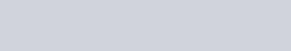The shaded part of the number line in given figure can also be represented as
Question:

The shaded part of the number line in given figure can also be represented as(a) $x \in\left(\frac{9}{2}, \infty\right)$

(b) $x \in\left[\frac{9}{2}, \infty\right)$

(c) $x \in\left[-\infty, \frac{9}{2}\right)$

(d) $x \in\left(-\infty, \frac{9}{2}\right]$

Solution:

Since shaded part includes $\frac{9}{2}$ and every point after $\frac{9}{2}$

$\therefore$ Shaded region can be represented by $\left[\frac{9}{2}, \infty\right)$

Hence, the correct answer is option B.